## Common plot types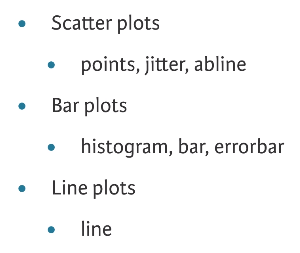## Grammatical Elements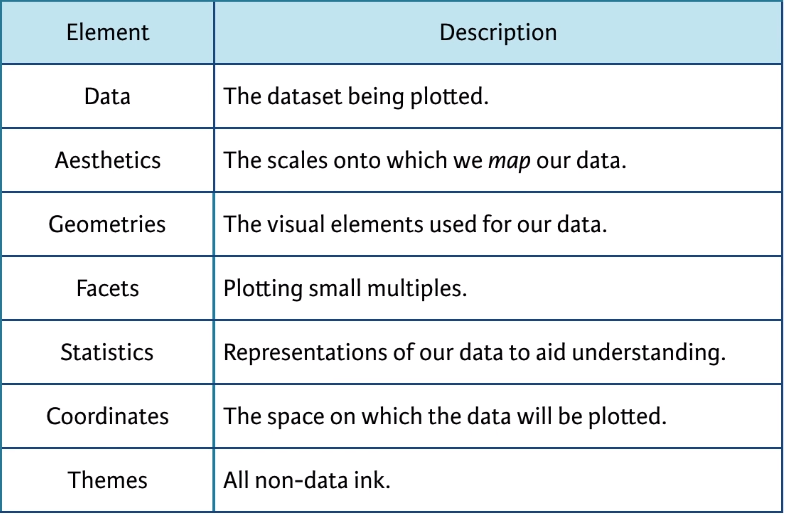## Aesthetics Arguments

Arguments of aes() : color, size, shape; Args of geom_points() : alpha (translucence)
``````library(ggplot2)
ggplot(mtcars, aes(x = factor(cyl), y = mpg)) + geom_point()
ggplot(mtcars, aes(x = wt, y = mpg, color = disp)) + geom_point()
ggplot(mtcars, aes(x = wt, y = mpg, size = disp)) + geom_point()

ggplot(diamonds, aes(x = carat, y = price)) +geom_point() + geom_smooth()
geom_smooth(method="auto", se=TRUE, fullrange=FALSE, level=0.95)``````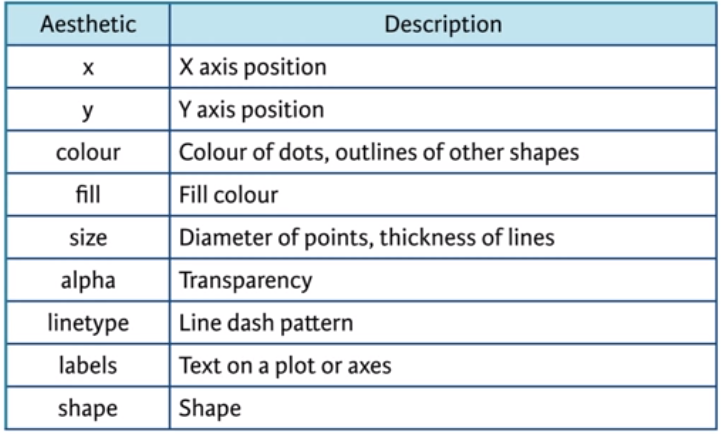The `color` aesthetic typically changes the outside outline of an object and the `fill` aesthetic is typically the inside shading. However, as you saw in the last exercise, `geom_point()` is an exception. Here you use `color`, instead of `fill` for the inside of the point. But it’s a bit subtler than that.

Which shape to use? The default `geom_point()` uses `shape = 19` (a solid circle with an outline the same colour as the inside). Good alternatives are `shape = 1` (hollow) and `shape = 16` (solid, no outline). These all use the `col` aesthetic (don’t forget to set `alpha` for solid points).

A really nice alternative is `shape = 21` which allows you to use both `fill` for the inside and `col` for the outline! This is a great little trick for when you want to map two aesthetics to a dot.

• method : smoothing method to be used. Possible values are lm, glm, gam, loess, rlm.
• method = “loess”: This is the default value for small number of observations. It computes a smooth local regression. You can read more about loess using the R code ?loess.
• method =“lm”: It fits a linear model. Note that, it’s also possible to indicate the formula as formula = y ~ poly(x, 3) to specify a degree 3 polynomial.
• se : logical value. If TRUE, confidence interval is displayed around smooth.
• fullrange : logical value. If TRUE, the fit spans the full range of the plot
• level : level of confidence interval to use. Default value is 0.95

Notice that mapping a categorical variable onto fill doesn’t change the colors, although a legend is generated! This is because the default shape for points only has a color attribute and not a fill attribute! Use fill when you have another shape (such as a bar), or when using a point that does have a fill and a color attribute, such as `shape = 21`, which is a circle with an outline. Any time you use a solid color, make sure to use alpha blending to account for over plotting.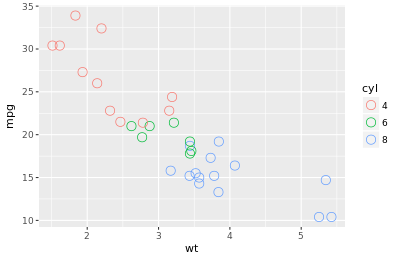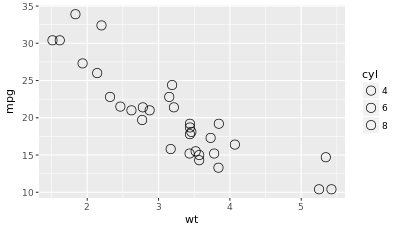```ggplot(mtcars, aes(x = wt, y = mpg, col = cyl)) + geom_point(shape = 1, size = 4) ```
``` ```
``` ```
``` ```
``` ```
``` ```
``` ggplot(mtcars, aes(x = wt, y = mpg, fill = cyl)) + geom_point(shape = 1, size = 4) ```
``` ```
``` ```
```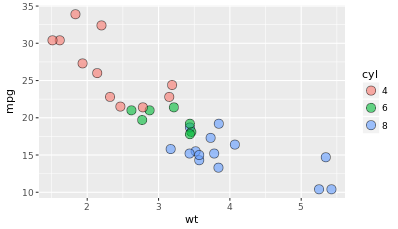ggplot(mtcars, aes(x = wt, y = mpg, fill = cyl)) + geom_point(shape = 21, size = 4, alpha=0.6) ggplot(mtcars, aes(x = wt, y = mpg, fill=cyl, col = am)) + geom_point(shape = 21, size = 4, alpha=0.6) ```
``` ```
``` ```
``` ```
``` ```
``` ```
```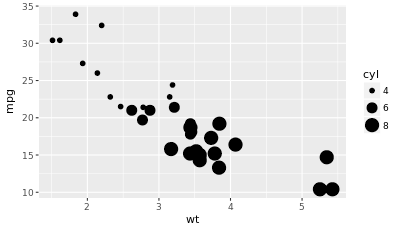ggplot(mtcars, aes(wt, mpg, size=cyl))+geom_point()Warning message: Using size for a discrete variable is not advised.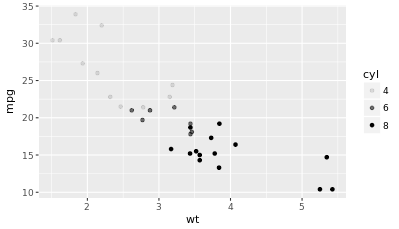ggplot(mtcars, aes(wt, mpg, alpha=cyl))+geom_point()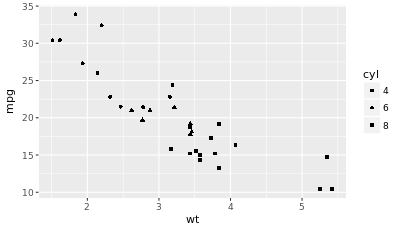ggplot(mtcars, aes(wt, mpg, shape = cyl))+geom_point()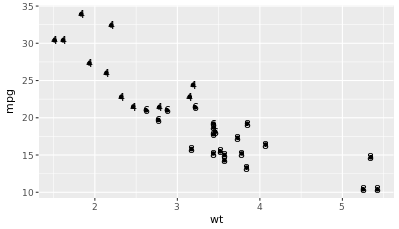ggplot(mtcars, aes(wt, mpg, label = cyl))+geom_point()+geom_text()ggplot(mtcars, aes(wt, mpg, size=cyl))+geom_point()Warning message: Using size for a discrete variable is not advised.ggplot(mtcars, aes(wt, mpg, alpha=cyl))+geom_point() Shapes in R can have a value from 1-25. Shapes 1-20 can only accept a color aesthetic, but shapes 21-25 have both a colorand a fill aesthetic. See the pch argument in par() for further discussion.A word about hexadecimal colours: Hexadecimal, literally “related to 16”, is a base-16 alphanumeric counting system. Individual values come from the ranges 0-9 and A-F. This means there are 256 possible two-digit values (i.e. 00 – FF). Hexadecimal colours use this system to specify a six-digit code for Red, Green and Blue values ("#RRGGBB") of a colour (i.e. Pure blue: "#0000FF", black: "#000000", white: "#FFFFFF"). R can accept hex codes as valid colours.Notice that if an aesthetic and an attribute are set with the same argument, the attribute takes precedence. Once again, you see that the attribute needs to match the shape and geom, the fill aesthetic (or attribute) will only work with certain shapes.label and shape are only applicable to categorical data. Attributes A scatter plot with color *aesthetic*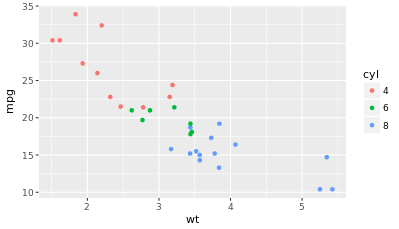ggplot(mtcars, aes(wt, mpg, col=cyl))+geom_point() Same, but set color *attribute* in geom layer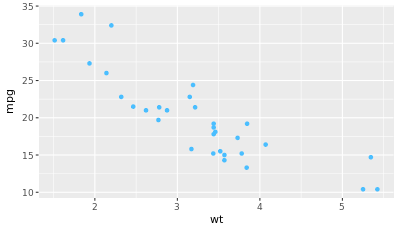ggplot(mtcars, aes(wt, mpg, col=cyl))+geom_point( col="#4ABEFF") Fill aesthetic; color, size and shape attributes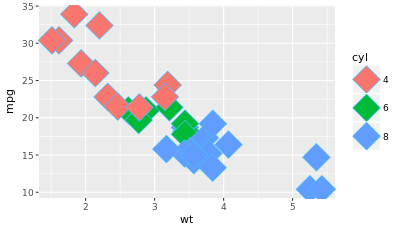ggplot(mtcars, aes(wt, mpg, fill=cyl))+geom_point( col=my_color, size=10, shape=23) Points with alpha 0.5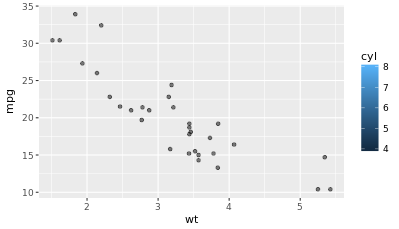ggplot(mtcars, aes(x = wt, y = mpg, fill = cyl))+geom_point(alpha=0.5) Points with shape 24 and color yellow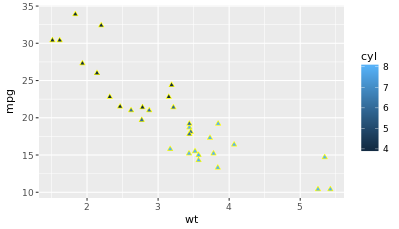ggplot(mtcars, aes(x = wt, y = mpg)) + geom_point(shape=24, col="Yellow") Fill aesthetic; color, size and shape attributesggplot(mtcars, aes(wt, mpg, fill=cyl))+geom_point( col=my_color, size=10, shape=23)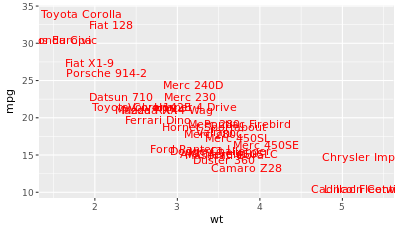ggplot(mtcars, aes(x = wt, y = mpg, fill = cyl))+geom_text(label=rownames(mtcars), col="red") The last plot displays five dimensions of the dataset: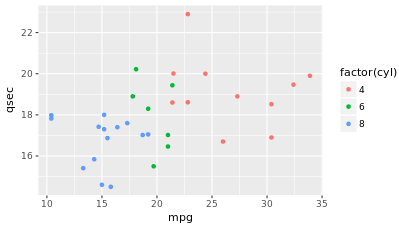ggplot(mtcars, aes(mpg,qsec,col=factor(cyl))) + geom_point()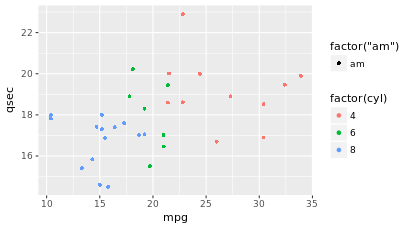ggplot(mtcars, aes(mpg,qsec,col=factor(cyl), shape=factor(am))) + geom_point()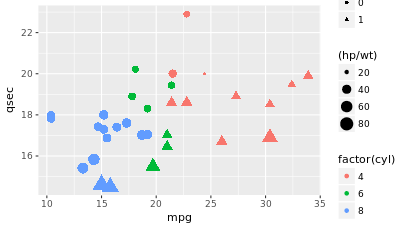ggplot(mtcars, aes(mpg,qsec,col=factor(cyl), shape=factor(am), size=(hp/wt))) + geom_point()Aesthetics for catigorical variables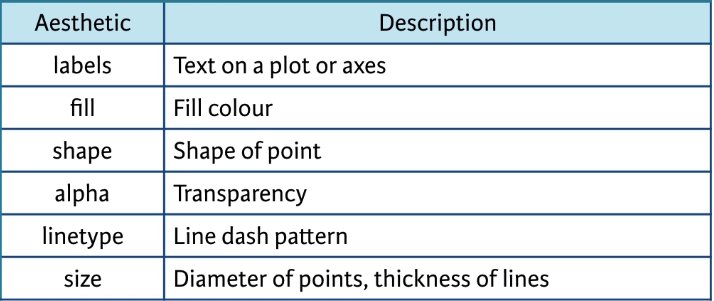Aesthetics for continuous variables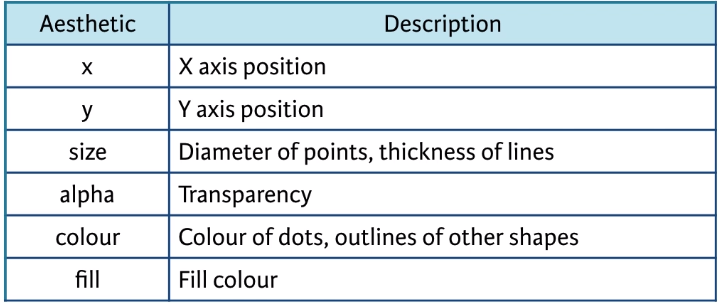Color is not the best choise for continuous scale aesthetic. Guide for categorical variablesQualitative colors are great for encoding nominal variablesSequential colors are better for ordinal variablesDirect labeling refers to actual group name on the plot.Hollow shapes are more easily distinguished than solid shapesCircles are always preferred to shapes with straight lines. Guide for continuous variables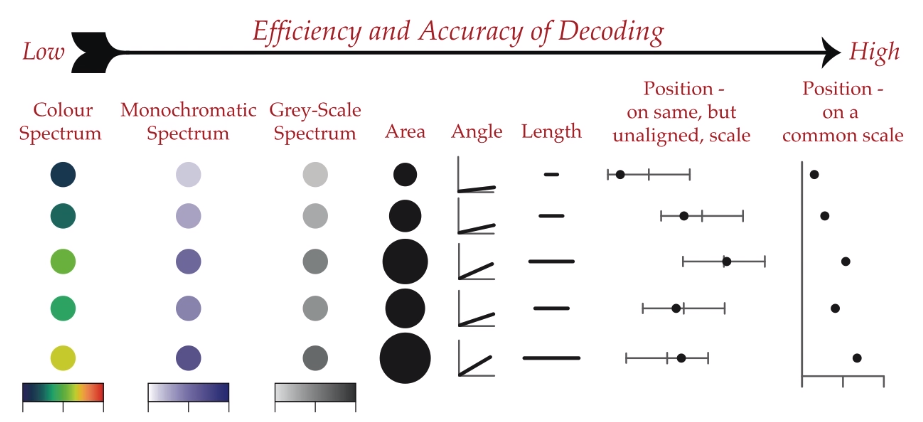Modifying Aesthetics Position specifies how ggplot will adjust for overlapping bars or points in a single layeridentity – default position in scatter plot – the value in the data frame is exactly where the value will be positioned in the plotdodgestackfilljitter – can be used as an argument, jitterdodge There is an issue with the precision in iris dataset. Sepals are measured to the nearest millimeter. We have 150 points and there is too much overplotting to distinguish them. To solve this, we need to add some random noise on both X and Y axes to to see regions of high density, which is referred to as jittering:ggplot(iris, aes(x=Sepal.Length, y=Sepal.Width, col=Species)) + geom_point(position="jitter")Jitter can be used as an argument, but each position type can also be accessed as a function, before the calling a plot:posn.j <- position_jitter(width = 0.1) ggplot(iris, aes(x=Sepal.Length, y=Sepal.Width, col=Species)) + geom_point(position=posn.j) This has two advantages: now we can set specific arguments for the position , such as the width , which defines how much random noise should be added, and it allow us to make use of this parameter throughout the plotting functions so that we can maintain consistency across plots.This is available to all position attributes. Each of the aesthetics is a scale which we mapped data onto, so color is just a scale, like X and Y. We can access all the scales with scale_ functions.scale_xscale_yscale_colorscale_fillscale_shapescale_linetypeAll the aesthetics have an associated scale function.We have to choose our axis depend of type of data e:scale_x_continuousscale_color_descreteThe first arg in scale function is always a scale, the second is one of these:limits – describe scales limitsbreaks – control the breaks on the guideexpand – numeric vector of length 2, giving a multiplicative and additive constant used to expand the ranges of the scales so that there is a small gap btw the data & the axeslabels – adjust the category namesTo quickly change the axis labels, use the lab functions.ggplot(iris, aes(x=Sepal.Length, y=Sepal.Width, col=Species))+ geom_point(position = "jitter")+ scale_x_continuous("Sepal Length")+ scale_color_discrete("Species") Scatter plots  are intuitive, easily understood and very common. A major consideration in any scatter plot is dealing with overplotting. You’ll have to deal with overplotting when you have:Large datasets,Imprecise data and so points are not clearly separated on your plot (you saw this in the video with the iris dataset),Interval data (i.e. data appears at fixed values), orAligned data values on a single axis.One very common technique that I’d recommend to always use when you have solid shapes it to use alpha blending (i.e. adding transparency). An alternative is to use hollow shapes. These are adjustments to make before even worrying about positioning.   mtcars\$cyl<-as.factor(mtcars\$cy)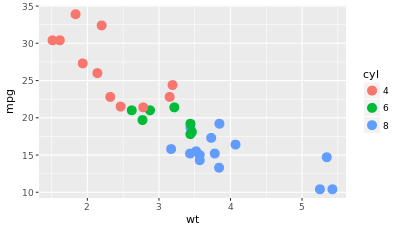# Basic scatter plot: # wt on x-axis and mpg on y-axis; #map cyl ggplot(mtcars, aes(x=wt, y=mpg, col=cyl)) + geom_point(size=4)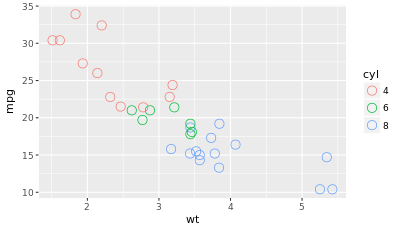# Hollow circles - an improvement ggplot(mtcars, aes(x=wt, y=mpg, col=cyl)) + geom_point(size=4, shape=1)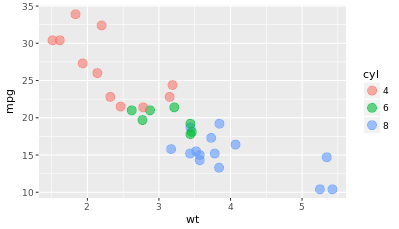# Add transparency - very nice ggplot(mtcars, aes(x=wt, y=mpg, col=cyl)) + geom_point(size=4, alpha=0.6) Dealing with large datasets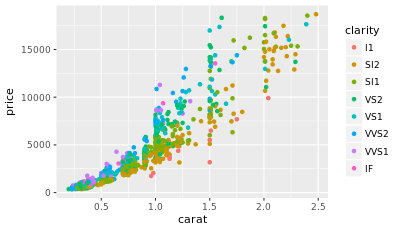#Basic scatter plot ggplot(diamonds, aes(x = carat, y = price, col = clarity)) +geom_point()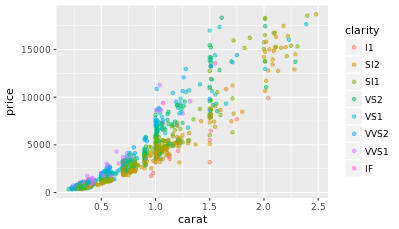# Adjust for overplotting, for large dataset ggplot(diamonds, aes(x=carat, y=price, col=clarity)) + geom_point(alpha=0.5)ggplot(diamonds, aes(x = clarity, y= carat, col=price)) + geom_point(alpha=0.5)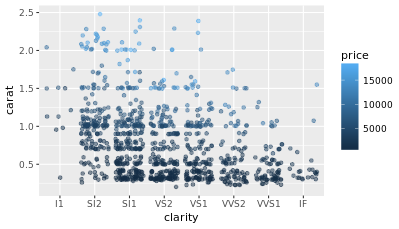# Dot plot with jittering ggplot(diamonds, aes(x = clarity, y= carat, col=price)) + geom_point(alpha=0.5, position="jitter") Geometries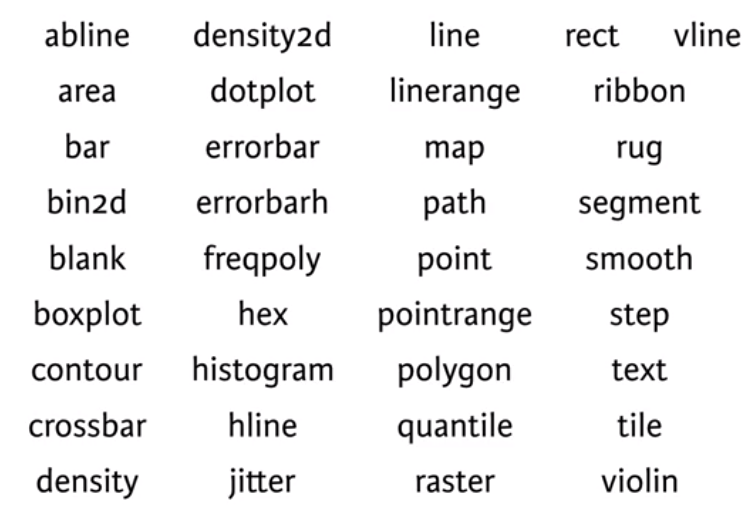Shape ~ pch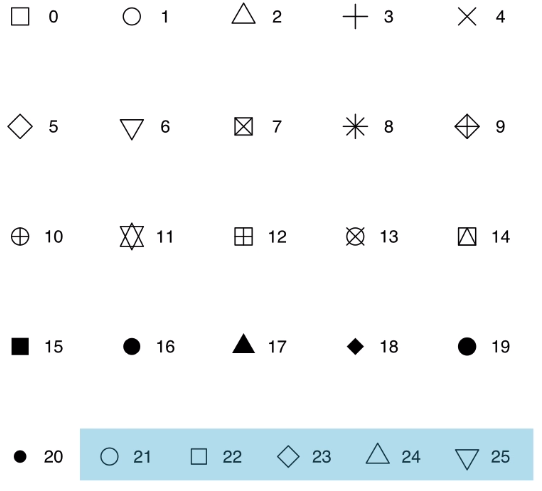* Shapes 21-25 have both fill & color, which can be controlled independently LinetypesPlot type Geometry Essential Optional Notes Scatter Plot geom_point() x, y alpha, color, fill, shape, size dots Content Content Content Content crosshairs marking where each mean value appears on the plot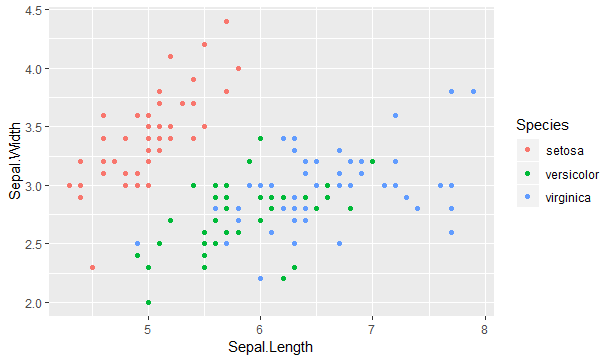ggplot(iris, aes(x=Sepal.Length, y=Sepal.Width, col=Species)) + geom_point()ggplot(iris, aes(x=Sepal.Length, y=Sepal.Width, col=Species)) + geom_point() + geom_point(data = iris.summary, shape = 15, size = 5) # 1st geom_point() inherits data & aes from ggplot # 2nd geom_point() is a different data * Shapes 21-25 have both fill & color, which can be controlled independently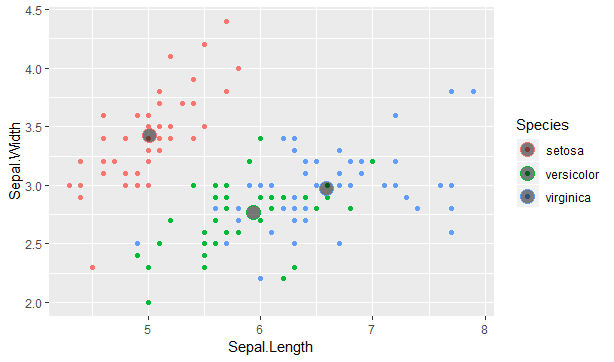ggplot(iris, aes(x=Sepal.Length, y=Sepal.Width, col=Species)) + geom_point() + geom_point(data = iris.summary, shape = 21, size = 5, fill='#00000080') Crosshairs marking where each mean value appears on the plot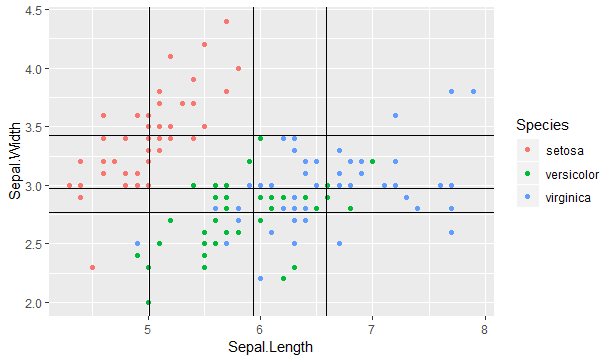ggplot(iris, aes(x=Sepal.Length, y=Sepal.Width, col=Species)) + geom_point() + geom_vline(data=iris.summary, aes(xintercept = Sepal.Length)) + geom_hline(data=iris.summary, aes(yintercept = Sepal.Width)) The color setting didn't get inherited, so we have to redefine it here: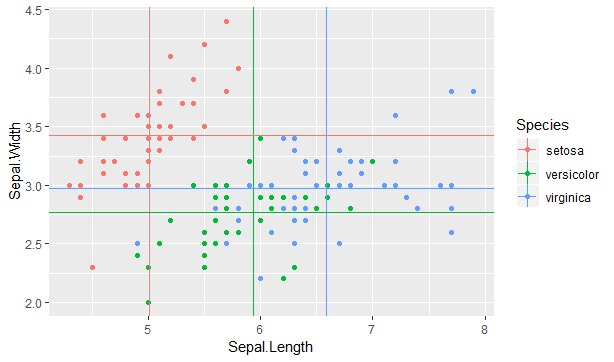ggplot(iris, aes(x=Sepal.Length, y=Sepal.Width, col=Species)) + geom_point() + geom_vline(data=iris.summary, aes(xintercept = Sepal.Length, col=Species, linetype=1)) + geom_hline(data=iris.summary, aes(yintercept = Sepal.Width, col=Species)) Jitter - helps tp see regions of high density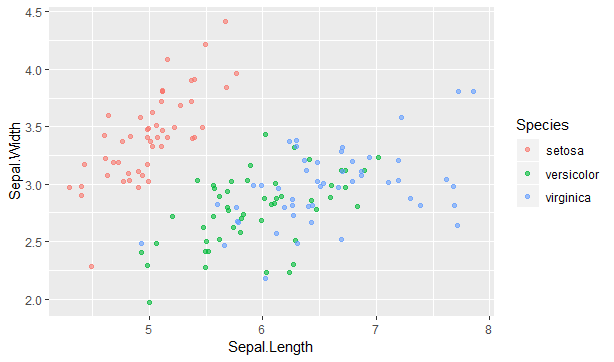ggplot(iris, aes(x=Sepal.Length, y=Sepal.Width, col=Species)) + geom_jitter(alpha=0.6) Another way - to change a symbol to a hollow cyrcle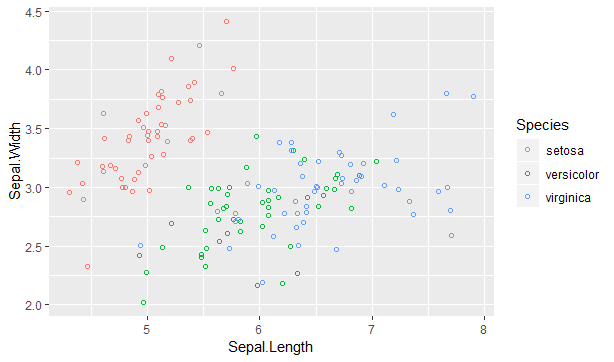ggplot(iris, aes(x=Sepal.Length, y=Sepal.Width, col=Species)) + geom_jitter(shape=1) Note: jittering adds some random noise to both axes. Recall: by changing the alpha, alpha & size, alpha & shape, we get a more detailed view of the data Barplots - Histogram, Bar, Errorbar Histogram Bars Type of data Numerical (quantities) Categorical (types, etc.)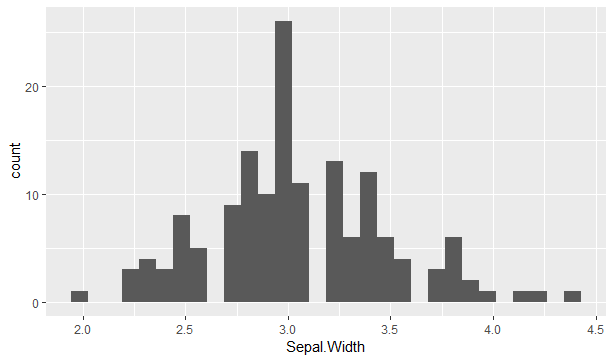ggplot(iris, aes(x=Sepal.Width)) + geom_histogram() diff(range(iris\$Sepal.Width))/30 = 0.8 ==> binwidth=0.8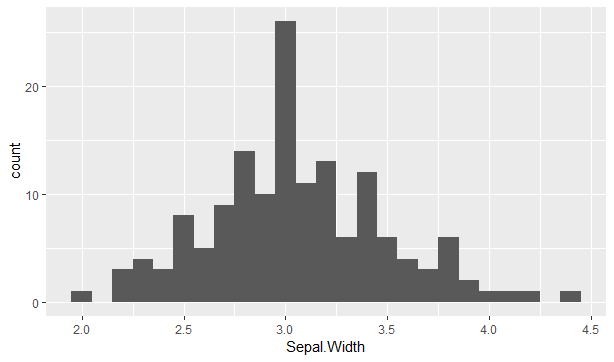ggplot(iris, aes(x=Sepal.Width)) + geom_histogram(binwidth=0.1) binwidth=0.1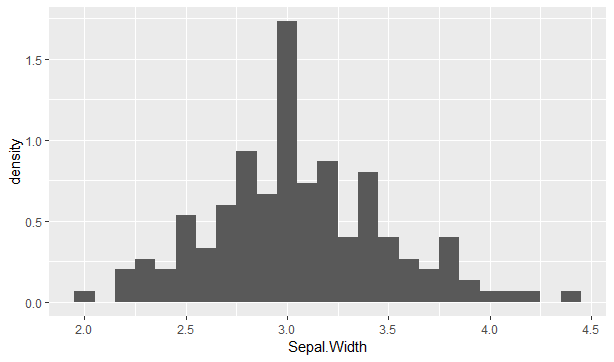ggplot(iris, aes(x=Sepal.Width)) + geom_histogram(binwidth=0.1)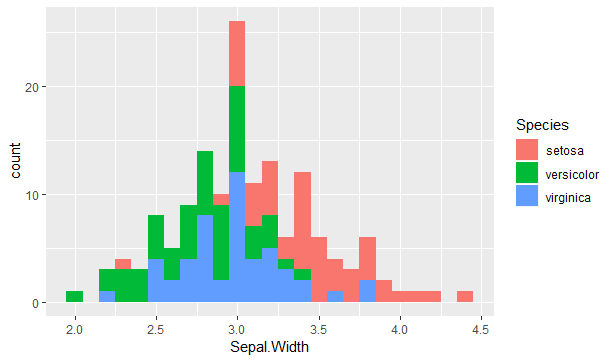ggplot(iris, aes(x = Sepal.Width, fill=Species)) + geom_histogram(binwidth=0.1)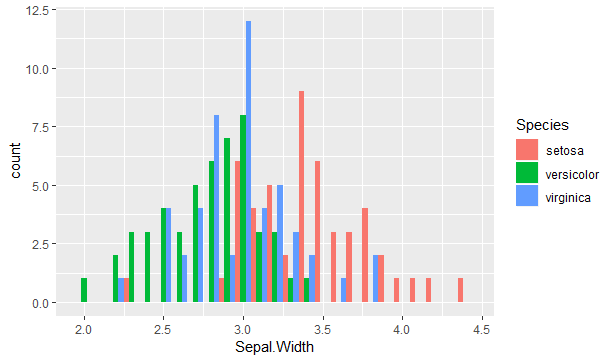ggplot(iris, aes(x=Sepal.Width, fill=Species)) + geom_histogram(binwidth=0.1, position="dodge") Histograms Histograms are one of the most common and intuitive ways of showing distributions. The x axis/aesthetic: geom_histogram()states the argument stat = "bin" as a default. Histograms cut up a continuous variable into discrete bins – that’s what the stat “bin” is doing. You always get 30 evenly-sized bins by default, which is specified with the default argument binwidth = range/30. The y axis/aesthetic: geom_histogram() only requires one aesthetic: x. But there is clearly a y axis on your plot, so where does it come from? Actually, there is a variable mapped to the y aesthetic, it’s called ..count… When geom_histogram() executed the binning statistic (see above), it not only cut up the data into discrete bins, but it also counted how many values are in each bin. So there is an internal data frame where this information is stored. The .. calls the variable count from this internal data frame. This is what appears on the y aesthetic. But it gets better! The density has also been calculated. This is the proportional frequency of this bin in relation to the whole data set. You use ..density.. to access this information.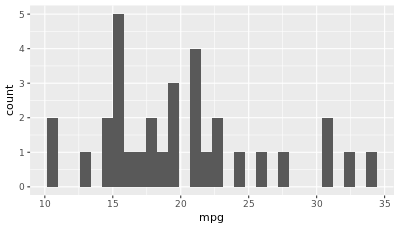ggplot(mtcars, aes(x = mpg)) + geom_histogram()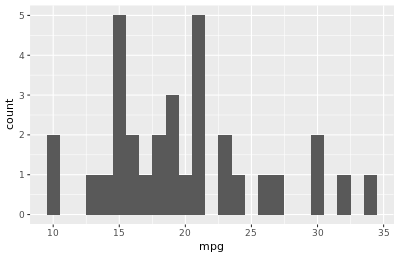ggplot(mtcars, aes(x = mpg)) + geom_histogram(binwidth=1)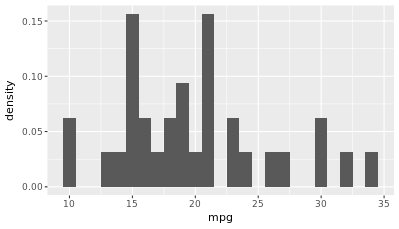ggplot(mtcars, aes(x = mpg)) + geom_histogram(aes(y=..density..),binwidth=1)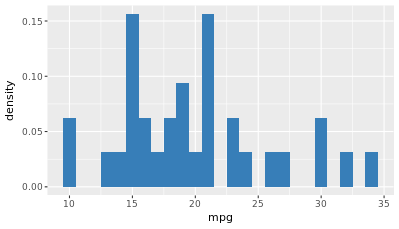ggplot(mtcars, aes(x = mpg)) + geom_histogram(fill="#377EB8",aes(y=..density..),binwidth=1) Frequency polygon is a unique solution for overlapping histograms. Frequency polygon plots, like kernel density plots, allow several distributions to be displayed in the same panel. This is a line connecting the value of each bin. Like geom_histogram(), it takes a binwidth argument. His defaults values are  stat = "bin", position = "identity". Position arguments for geom_bar(), geom_histogram()stack: [default] place the bars on top of each other. Counts are used. This is the default position.fill: [proportion] place the bars on top of each other, but this time use proportions.dodge: place the bars next to each other. Counts are used. mtcars\$cyl <- as.factor(mtcars\$cyl) mtcars\$am <- as.factor(mtcars\$am)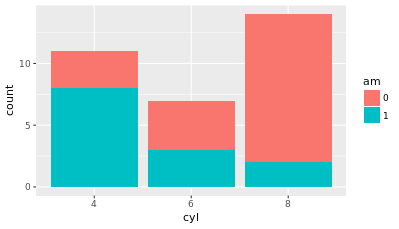ggplot(mtcars, aes(x = cyl, fill = am)) + geom_bar() ggplot(mtcars, aes(x = cyl, fill = am)) + geom_bar(position="stack")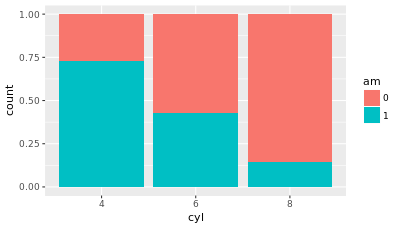ggplot(mtcars, aes(x = cyl, fill = am)) + geom_bar(position="fill")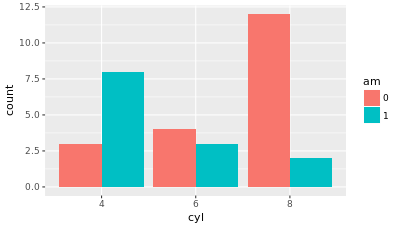ggplot(mtcars, aes(x = cyl, fill = am)) + geom_bar(position="dodge") Adjusting the dodging position = “dodge” –> position_dodge() The reason you want to use position_dodge() (and position_jitter()) is to specify how much dodging (or jittering) you want. mtcars\$cyl <- as.factor(mtcars\$cyl) mtcars\$am <- as.factor(mtcars\$am)ggplot(mtcars, aes(x = cyl, fill = am)) + geom_bar() ggplot(mtcars, aes(x = cyl, fill = am)) + geom_bar(position="stack")ggplot(mtcars, aes(x = cyl, fill = am)) + geom_bar(position="fill")ggplot(mtcars, aes(x = cyl, fill = am)) + geom_bar(position="dodge")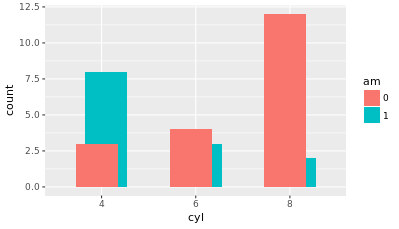posn_d <- position_dodge(width=0.2) ggplot(mtcars, aes(x = cyl, fill = am)) + geom_bar(position = posn_d)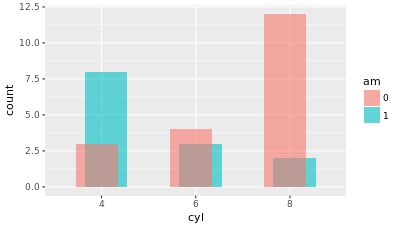posn_d <- position_dodge(width=0.2) ggplot(mtcars, aes(x = cyl, fill = am)) + geom_bar(position = posn_d, alpha=0.6)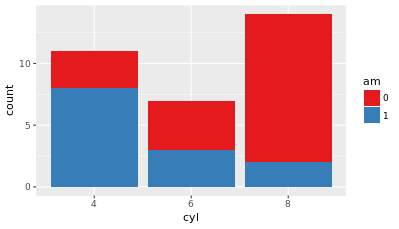# Example of how to use a brewed color palette ggplot(mtcars, aes(x = cyl, fill = am)) + geom_bar() + scale_fill_brewer(palette = "Set1") Overlapping: histogram & bars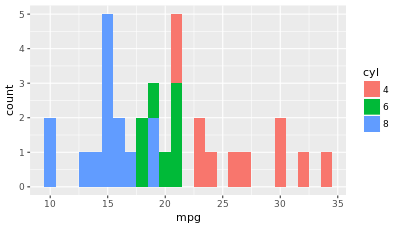ggplot(mtcars, aes(mpg, fill=cyl)) + geom_histogram(binwidth = 1)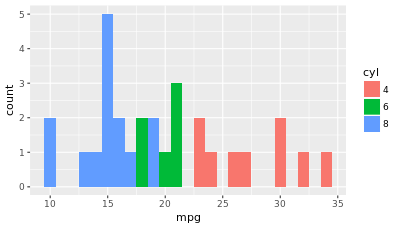ggplot(mtcars, aes(mpg, fill=cyl)) + geom_histogram(binwidth = 1, position="identity")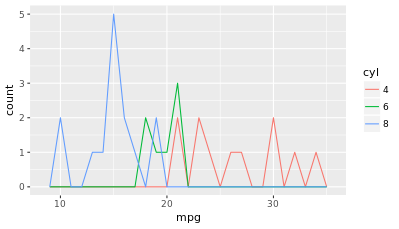ggplot(mtcars, aes(mpg, col=cyl)) + geom_freqpoly(binwidth = 1) #position is "identity" by default Bar plots with color ramp Vocab\$education <- as.factor(Vocab\$education) Vocab\$vocabulary <- as.factor(Vocab\$vocabulary)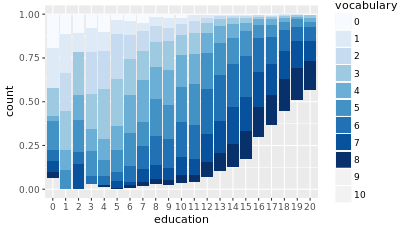ggplot(Vocab, aes(x=education, fill=vocabulary)) + geom_bar(position="fill") + scale_fill_brewer()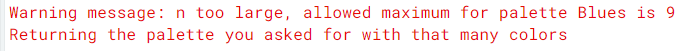This is an incomplete bar plot. This was because for continuous data, the default RColorBrewer palette that scale_fill_brewer() calls is “Blues”. There are only 9 colours in the palette, and since we have 11 categories, the plot looks strange.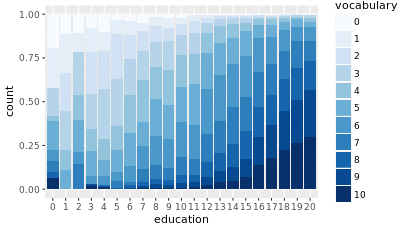# Definition of a set of blue colors blues <- brewer.pal(9, "Blues") # from the RColorBrewer package # Making a color range using colorRampPalette() and the set of blues blue_range <- colorRampPalette(blues) ggplot(Vocab, aes(x = education, fill = vocabulary)) + geom_bar(position = "fill") + scale_fill_manual(values = blue_range(11)) new_col <- colorRampPalette(c("#FFFFFF", "#0000FF")) new_col(4) # the newly extrapolated colours munsell::plot_hex(new_col(4)) # Quick and dirty plot #new_col() is a function that takes one argument: the number of colours you want to extrapolate. Overlapping solution for multiple histograms, as long as there are not too many different overlaps!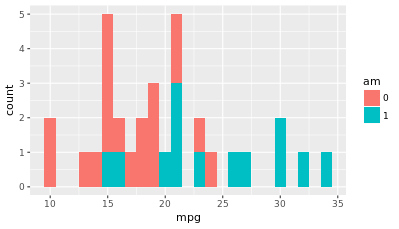ggplot(mtcars, aes(mpg, fill=am)) + geom_histogram(binwidth = 1) # position="stack" by default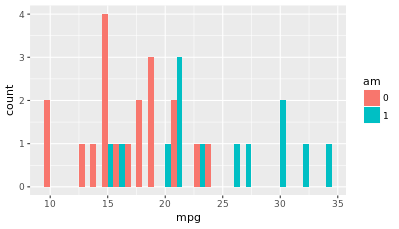ggplot(mtcars, aes(mpg, fill=am)) + geom_histogram(binwidth = 1, position="dodge")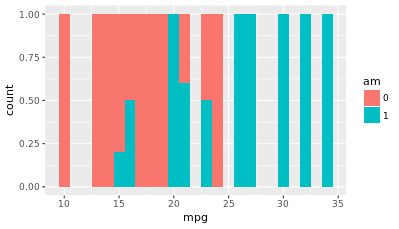ggplot(mtcars, aes(mpg, fill=am)) + geom_histogram(binwidth = 1, position="fill") In this case, none of these positions really work well, because it's difficult to compare the distributions directly.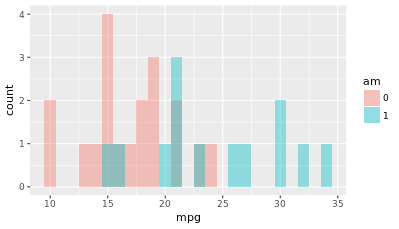ggplot(mtcars, aes(mpg, fill=am)) + geom_histogram(binwidth = 1, position="identity", alpha=0.4)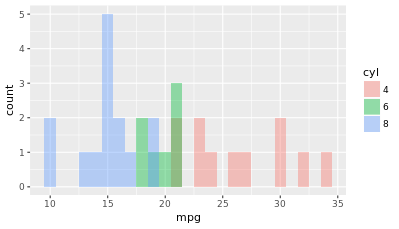ggplot(mtcars, aes(mpg, fill=cyl)) + geom_histogram(binwidth = 1, position="identity", alpha=0.4) Time Series Series can be encoded usingline type – dashes,size – sickness,color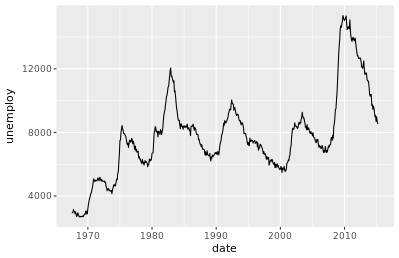# Plot unemploy as a function of date # using a line plot ggplot(economics, aes(x = date, y = unemploy)) + geom_line()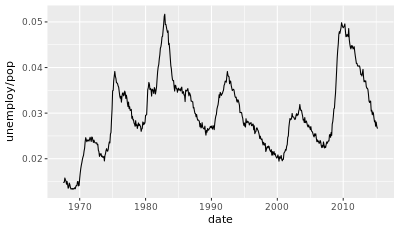# Adjust plot to represent the fraction # of total population that is unemployed ggplot(economics, aes(x = date, y = unemploy/pop)) + geom_line()There is a large spike in unemployment during recession periods. # geom_rect() to draw the recess periods ggplot(economics, aes(x = date, y = unemploy/pop)) + geom_rect(data = recess, aes(xmin = begin, xmax = end, ymin = -Inf, ymax = +Inf), inherit.aes = FALSE, fill = "red", alpha = 0.2) + geom_line()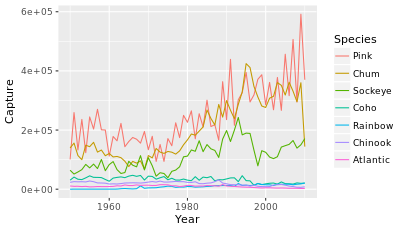fish.tidy <- gather(fish.species, Species, Capture, -Year) ggplot(fish.tidy, aes(x = Year, y = Capture, col=Species)) + geom_line() ```
``` ```
``` ```
``` ```
``` Recent Posts WordPress Resources at SiteGround Hello world! Archives June 2018 June 2020 M T W T F S S « Jun     1234567 891011121314 15161718192021 22232425262728 2930   ©2020 * WebMust /* <![CDATA[ */ var localize = {"ajaxurl":"https:\/\/webmust.org\/wp-admin\/admin-ajax.php"}; /* ]]> */ var ElementorProFrontendConfig = {"ajaxurl":"https:\/\/webmust.org\/wp-admin\/admin-ajax.php","nonce":"bd472587bb","shareButtonsNetworks":{"facebook":{"title":"Facebook","has_counter":true},"twitter":{"title":"Twitter"},"google":{"title":"Google+","has_counter":true},"linkedin":{"title":"LinkedIn","has_counter":true},"pinterest":{"title":"Pinterest","has_counter":true},"reddit":{"title":"Reddit","has_counter":true},"vk":{"title":"VK","has_counter":true},"odnoklassniki":{"title":"OK","has_counter":true},"tumblr":{"title":"Tumblr"},"delicious":{"title":"Delicious"},"digg":{"title":"Digg"},"skype":{"title":"Skype"},"stumbleupon":{"title":"StumbleUpon","has_counter":true},"telegram":{"title":"Telegram"},"pocket":{"title":"Pocket","has_counter":true},"xing":{"title":"XING","has_counter":true},"whatsapp":{"title":"WhatsApp"},"email":{"title":"Email"},"print":{"title":"Print"}},"facebook_sdk":{"lang":"en_US","app_id":""}}; var elementorFrontendConfig = {"environmentMode":{"edit":false,"wpPreview":false},"is_rtl":false,"breakpoints":{"xs":0,"sm":480,"md":768,"lg":1025,"xl":1440,"xxl":1600},"version":"2.5.16","urls":{"assets":"https:\/\/webmust.org\/wp-content\/plugins\/elementor\/assets\/"},"settings":{"page":[],"general":{"elementor_global_image_lightbox":"yes","elementor_enable_lightbox_in_editor":"yes"}},"post":{"id":865,"title":"ggplot2","excerpt":""}}; ```